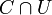# Resolvability is open subspace-closed

This article gives the statement, and possibly proof, of a topological space property (i.e., resolvable space) satisfying a topological space metaproperty (i.e., open subspace-closed property of topological spaces)
View all topological space metaproperty satisfactions | View all topological space metaproperty dissatisfactions
Get more facts about resolvable space |Get facts that use property satisfaction of resolvable space | Get facts that use property satisfaction of resolvable space|Get more facts about open subspace-closed property of topological spaces

## Statement

Suppose$X$ is a resolvable space and$U$ is an open subset of$X$. Then,$U$ is a resolvable space with the subspace topology.

## Facts used

1. Intersection of dense subset with open subset is dense in the open subset

## Proof

Given: A topological space$X$ with disjoint dense subsets$C$ and$D$. An open subset$U$ of$X$.

To prove:$U$ has two disjoint dense subsets.

Proof:

Step no. Assertion/construction Facts used Given data used Previous steps used Explanation
1$C \cap U$ and$D \cap U$ are both dense in$U$. Fact (1)$C,D$ dense in$X$$U$ open in$X$
given-fact direct
2$C \cap U$ and$D \cap U$ are disjoint.$C,D$ are disjoint basic set theory!
3$C \cap U$ and$D \cap U$ are the desired disjoint dense subsets in$U$ Steps (1), (2) Step-combination direct